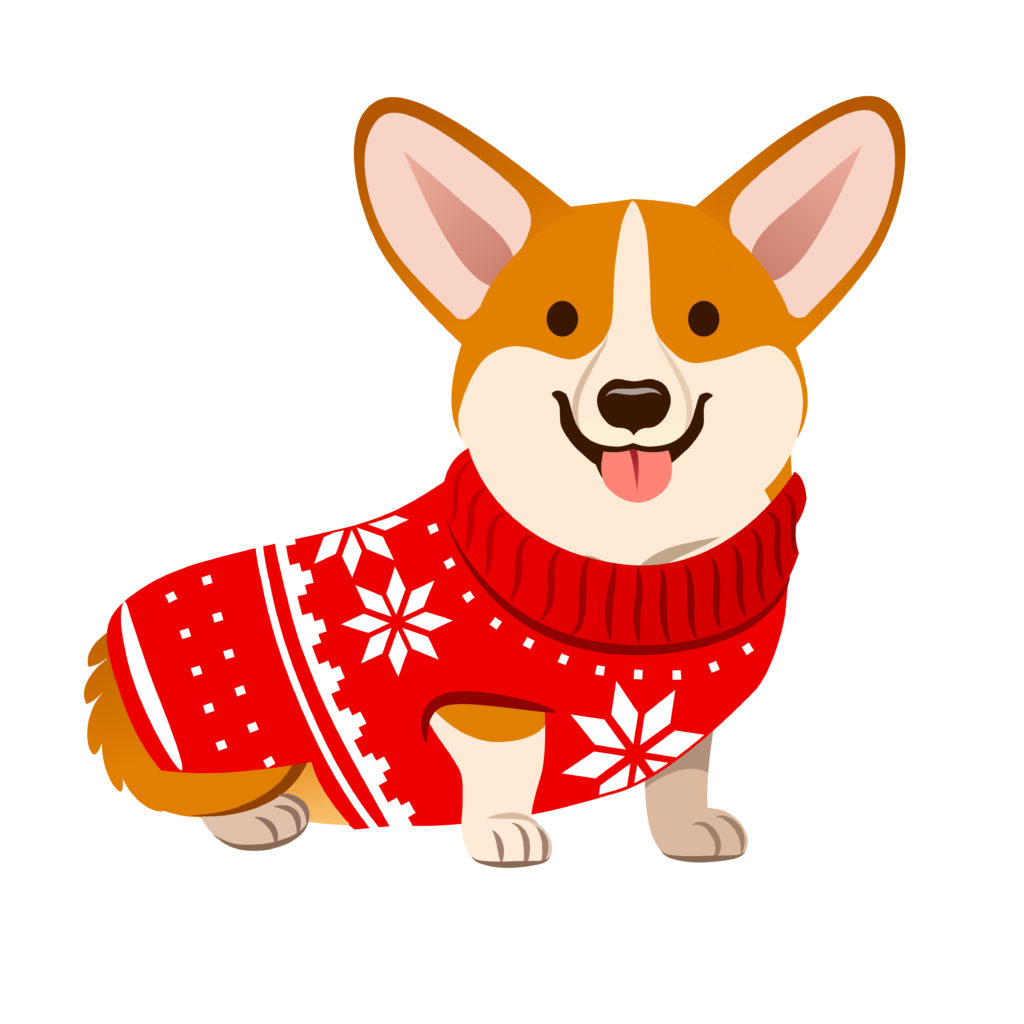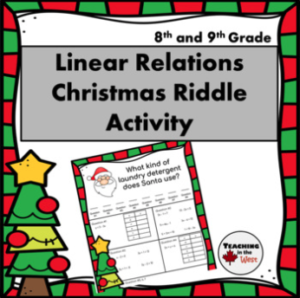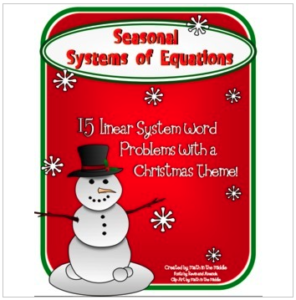Fight the holiday brain drain with these fun and engaging free Christmas math activities to incorporate into your classroom this holiday season. From hidden messages and color-by-numbers to linear systems and word problems, you’ll find no shortage of mathematical in this Yuletide spread.

We’ve also included some unique Piqosity Christmas word problems at the end of this post to put your holiday math knowledge to the test!

## Christmas Math Activities for Middle Schoolers

###Linear Relations Christmas Riddle

The math riddle students need to solve in this printable Christmas activity will challenge your students to solve equations, make tables with different values, and select graph points for equations too. The worksheet includes seven different questions for students to solve, along with a hidden letter that will correspond with their answers.

This is a great activity to do individually or in pairs and makes the perfect activity for review at the beginning of class.

## Christmas Math Activities for High Schoolers

###Seasonal Linear System Word Problems

This free Christmas math activity is a printable worksheet that requires students to solve 15 different word problems. Each word problem answer will give them an ordered pair that they will need to plot on a linear graph. Once they’ve answered all 15 questions and graphed each pair, they’ll connect the dots to reveal a hidden holiday picture. This free download comes with two pages of word problems, an empty linear graph to fill out, and an answer key for you to compare student answers to.

Hidden picture graphs are a great way to get students excited about learning and practicing plotting ordered pairs correctly.

### Algebra II Color By Numbers

These Algebra II Color By Number freebies give you the option to use one for practice the Pythagorean Theorem, special right triangles, trigonometry, and the law of sines. Each concept is a different Christmas-themed printable.

Each printable comes with a color-by-number picture, word problem pages, and a convenient answer key to help you check over student work. This is a great activity to assign students in the week leading up to winter break. This way, students get to celebrate the holiday without sacrificing valuable lesson time!

## Free Christmas Math Questions by Piqosity

Even Christmas elves have to do math sometimes! Piqosity devised free Christmas math problems, starting at a 5th-grade level and progressing in difficulty to Algebra 1, in order to test your math knowledge and get you and your students into the holiday spirit.

These problems, written by Piqosity’s own Mohamed El Noamany, are easy to copy/paste and turn into your own Christmas math worksheet!

### Christmas Math Questions

1. In the North pole, half of all the elves are assigned present building duty and one-third are assigned wrapping duty. If an eighth of all the elves are assigned to look after the reindeer and the rest are unemployed, what fraction of the total elves do not have a job?

A.B.C.D.2. A store is having a Christmas sale of 35% off on Santa Claus costumes. If the original cost of each costume is $350, how much will it cost after the sale? A.$227.50
B. $210.00 C.$245.00
D. $192.50 3. “The Twelve Days of Christmas” describes a lucky person who gets one additional gift each day for 12 days, starting with 1 gift on the first day. What is the total number of gifts that the lucky person receives after the twelfth day? A. 78 B. 66 C. 144 D. 72 ### Math Answer Key and Explanations 1. B In order to find the fraction of unemployed elves, we need to first find the total fraction of elves with jobs and then subtract the result from 1.In order to add these fractions, we will need to find the least common multiple that the denominators share. In this case, the least common multiple of 2, 3 and 8 is 24.Subtract this fraction from 1.The total fraction of unemployed elves in the North Pole is. 2. A Since we are marking down the price of the costume by 35%, we can calculate the new price of the costume by multiplying the original price by 0.65. 350 × 0.65 = 227.50 The new cost of the Santa costume is$227.50.

3. A

Here, we are trying to find the sum of all the numbers from 1-12. To find the sum of a sequence of numbers, we can use the following formula:Where n is the number of terms in the sequence, a is the first term in the sequence and a is the last term in the sequence.

In this case, we have 12 terms so n is equal to 12, a is equal to 1 and a is equal to 12.

Plug these values in to solve for the sum.## Find More Math Resources Like These at Piqosity!

We hope that these free Christmas math activities were filled with enough Christmas magic to keep your students engaged (and Frosty “all livin’!”) You can find more (non-Christmas-themed) Math lessons with questions of similar difficulty levels in our ELA and Math Courses! These are complete courses available online through our app and can be purchased separately or received for free when bundled with our ISEE test prep courses!

For your convenience, we have outlined lessons relevant to or at the same difficulty level as the above word problems. These can be found below.

### Related Math Lessons by Piqosity:

Lessons related to question #1:

Lessons related to question #2:

Lessons related to question #3:

If you enjoyed these Christmas-themed math activities, don’t forget to check out our FREE Christmas reading activities, too!